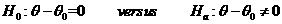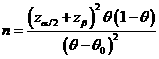# Sample Size Calculator: One Sample Proportion

Hypothesis: Two-Sided EqualityData Input: (Help) (Example)

 Input Results α β N θ θ0

Note:

 Variables Descriptions α Two-sided significance level 1-β Power of the test θ Expected success proportion of sample θ0 Known success proportion N Sample size

Help Aids

Application: To test whether there is a difference between the mean response rates of the test drug and the reference drug and the following hypotheses are usually used:Procedure:

1. Enter

a)      value of α, the probability of type I error

b)      value of β, the probability of type II error

c)      value of θ, a true mean response rate of a test drug

d)     value of θ0, a reference response rate

1. Click the button “Calculate” to obtain result sample size N.

Formula:(*)

Notations:

α:               The probability of type I error (significance level) is the probability of rejecting the true null hypothesis.

β:               The probability of type II error (1 – power of the test) is the probability of failing to reject the false null hypothesis.

θ-θ0:          The difference between the true mean response rates of a test drug (θ) and a reference value (θ0).

Examples

Example 1: Suppose the response rate of the patient population under study after treatment is expected to be around 50% (i.e., θ=0.5). By (*), at α=0.05, the required sample size for achieving an 80% power (β=0.2) correctly detecting a difference between the post-treatment response rate and the reference value say, 30% (i.e., θ0=0.3) is N=50.

Reference:

1. Casagrande, Pike and Smith (1978), Biometrics 34: 483-486.
2. Chow, Shao and Wang, Sample Size Calculations In Clinical Research, Taylor & Francis, NY. (2003) Pages 82-83.
3. Flesis J.L., Statistical Methods for Rates and Proportions (2nd edition). Wiley: New York, 1981.